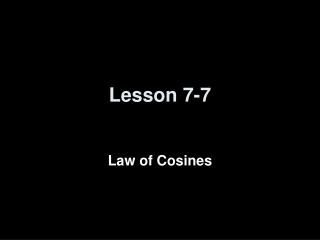DownloadDownload PresentationLesson 7-7

# Lesson 7-7

Télécharger la présentation## Lesson 7-7

- - - - - - - - - - - - - - - - - - - - - - - - - - - E N D - - - - - - - - - - - - - - - - - - - - - - - - - - -
##### Presentation Transcript

1. Lesson 7-7 Law of Cosines

2. Transparency 7-7 5-Minute Check on Lesson 7-6 • Find each measure given the measures of ∆RST. Round all side measurements to the nearest tenth and angles to the nearest degree.. • Find s, if mR = 63°, mS = 38°, and r = 52. • Find mR, if mS = 122°, s = 10.8, and r = 5.2. • Solve ∆MNP described below. Round all side measurements to the nearest tenth and angles to the nearest degree. • mM = 50°, if mN = 32°, and m = 15. • n = 8.5, p = 10.8, and mP = 110°. • Find the perimeter of quadrilateral ABCD to the nearest tenth. 35.9 24° mP = 98°, n = 10.4, p = 19.4 mN = 48°, mM = 22°, m = 4.4 Standardized Test Practice: 70° 8 cm 54° B 27.6 29.8 32.0 34.6 A B C D Click the mouse button or press the Space Bar to display the answers.

3. Objectives • Use the Law of Cosines to solve triangles • Solve problems by using the Law of Cosines

4. Vocabulary • None new

5. Law of Cosines A Let ∆ABC be any triangle with a, b and c representing the measures of the sides opposite the angles with measures A, B, and C respectively. Then the following equations are true: b c C B a a2 = b2 + c2 – 2bc cos A b2 = a2 + c2 – 2ac cos B c2 = a2 + b2 – 2ab cos C Law of Cosines can be used to solve triangles when the Law of Sines cannot be used Case 1: measures of two sides and their included angle (SAS) Case 2: measures of all three sides (SSS)

6. Answer: Example1 Use the Law of Cosines since the measures of two sides and the included angle are known. Law of Cosines Simplify. Take the square root of each side. Use a calculator.

8. Answer: Example 3 Law of Cosines Simplify. Subtract 754 from each side. Divide each side by –270. Solve for L. Use a calculator.